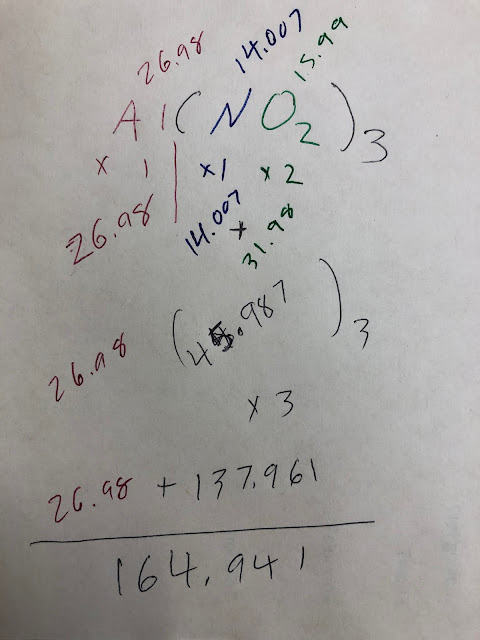## Sunday, October 18, 2020

### Chemical Notation to Molecular Mass

General Chemistry Index

Where are we going with this? This page will give the ability to demonstrate an understanding of the law of conservation of mass through the use of particle diagrams and mathematical models.

Chemical Notation to Molecular Mass
This is actually pretty easy. And sort of cool, in a sciency way.

Beginning with the correct notation for a particular element or compound is the first step in doing all this stoichiometry stuff!

The good news is that if you have a balanced chemical reaction, you have the correct notation… you have what you need.

So, we need to figure out how much one mole of a thing is… Good thing we have that periodic table and those atomic masses!

The atomic weight of an element indicates the mass in grams of one mole of those atoms. Bam!https://ptable.com/#Properties
So, look at potassium:

The number at the bottom of the element listing is the atomic mass. That number, for potassium, is 39.098.

That means that one mole of potassium has a mass of 39.098 grams. Or if your scale only goes to the .01 accuracy, 39.10 grams.

So, let's go with this and see what happens!

Suppose you have the reaction of K and O2 such that:

4K + O2 --> 2K2O

So, in this step we are answering this question:

What is the mass in grams of each of the molecules in the reaction?

We are NOT asking about total grams. Not in this step. Just the mass of ONE molecule. We are finding the molecular weight. How about a definition?

Molecular weight (analogous to atomic weight) is the mass in grams of one mole of molecules of a substance.

To find molecular weight, add up all of the atomic weights of all the atoms in the molecule.

Let's do that! Could be fun!

In the reaction:

4K + O2 --> 2K2O

we have three molecules (one of which is an element):

K
O2
K2Ohttps://ptable.com/#Properties

We already looked up K, so we know that one mole of K weighs 39.098 grams.

Now, for that O2

Each molecule has two oxygen atoms.

Using the periodic table again, once more looking at the atomic weight, we find that one atom of oxygen has a mass of 15.999 grams.

So, that's the mass of one atom. Now, how many atoms are in each oxygen molecule?

Two (aka 2).

Because it is O2.

So, the mass of the molecule is the mass of the atom times however many atoms there are. Therefore, the mass of one molecule of O2 is found as

15.999 X 2 = 31.998

So, the mass of one molecule of oxygen is 31.998 grams.

Told you it was kinda cool…

Now, we have one more molecule to calculate. The K2O one…

This guy has more than one type of atom in it. But, no biggie. We just add!

K2O

Two K and one O atoms.

So, to be mathy about it, the mass of one K2O is:

K2O[mass]  = 2 • K[mass] + O[mass]
K2O[mass]  = 2 • 39.098 gr + 15.999 gr
K2O[mass]  = 2 • 39.098 gr + 15.999 gr
K2O[mass]  = 94.179 grams

________________________________________________________________
Doing it that way looks awful. How about doing it with the chemical reaction? Could it be worse?

4K + O2 --> 2K2O

First off, we are only looking at the mass of the molecules, so we don't in this case need the coefficients.

So we're going to more or less ignore the coefficients:

4K + O2 --> 2K2O

Next, put the atomic masses above each of the atoms. (And spread it out, because you need room.)

39.098            15.999                39.098    |  15.999

4K          +          O2        -->                   2K2O

Next, multiply the atomic mass by the subscript in the molecule:

39.098            15.999                39.098      |     15.999

4K          +        O         -->                2K2O

39.098            15.999                39.098      |     15.999

X 1                X 2                    X 2      |         X 1
_______          ______            ________        _____
39.098            31.998                78.196      +     15.999 <--Add the two parts of the molecule.)

94.195

So, doing that, you now have the molecular weights for each part of the reaction.

If you have very complex molecules (such as polyatomics), the process is the same. Just more steps.Molecular Mass of Al(NO2)3 with some rounding.